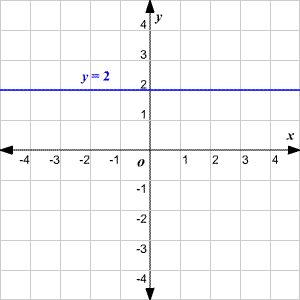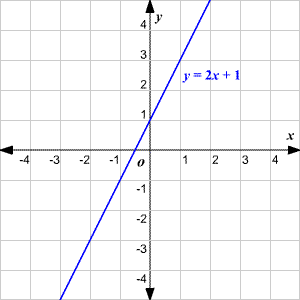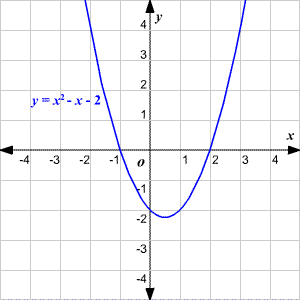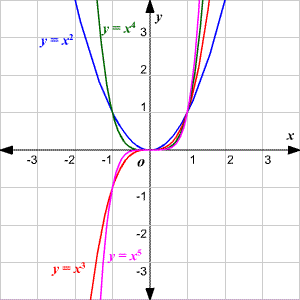# Graphing Functions of the form ${x}^{n}$

A polynomial function is a function in which $f\left(x\right)$ is a polynomial in $x$ .

A polynomial function of degree $n$ is written as $f\left(x\right)={a}_{n}{x}^{n}+{a}_{n-1}{x}^{n-1}+{a}_{n-2}{x}^{n-2}+...+{a}_{2}{x}^{2}+{a}_{1}x+{a}_{0}$ .

The graph of a polynomial function of degree $0$ , which is of the form $f\left(x\right)=a$ is a horizontal line .The graph of a polynomial function of degree $1$ , which is of the form $f\left(x\right)=ax+b,\text{\hspace{0.17em}}\text{\hspace{0.17em}}a\ne 0$ , is a non-horizontal line. Read more about graphing lines here .The graph of a polynomial function of degree $2$ , also called a quadratic equation , which is of the form $f\left(x\right)=a{x}^{2}+bx+c,\text{\hspace{0.17em}}\text{\hspace{0.17em}}a\ne 0$ , is a parabola. Read more about graphing parabolas here .Higher-degree polynomials can be somewhat more difficult to graph. Some easy cases are the parent graphs $f\left(x\right)={x}^{3},f\left(x\right)={x}^{4},f\left(x\right)={x}^{5}$ , etc. These graphs have a predictable shape depending on whether the exponent is even or odd, as shown below.Other higher-degree polynomials with more terms require other techniques. One method is to factor the polynomials to find $x$ -intercepts.

You can also use calculus to find the critical points of a polynomial function.